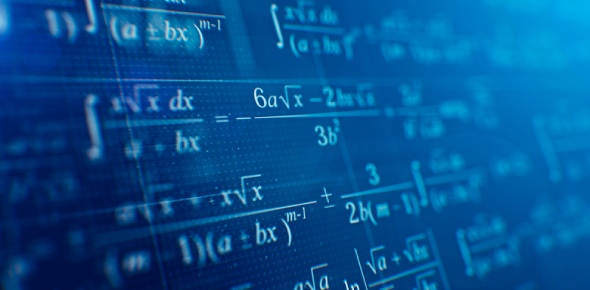# Timed Mental Mathematics #2

7 Questions | Total Attempts: 49SettingsMore questions to be answered within five minutes. Good luck!

Related Topics
• 1.
2+(21z)=65 z=
• A.

4

• B.

2

• C.

3

• D.

5

• 2.
For the following equation, c=12.35(2)+c=zz=
• A.

82

• B.

79

• C.

84

• D.

85

• 3.
Which of the following choices are equal to 36?
• A.

78-38

• B.

12+27

• C.

53-17

• D.

17(2)

• 4.
Which of the following choices are equal to 45?
• A.

6(9)

• B.

23(2)

• C.

80-35

• D.

17+36

• 5.
True or false?98+43-104(2)=78
• A.

True

• B.

False

• 6.
True or false?59(6)=354
• A.

True

• B.

False

• 7.
-55+23=
• A.

24

• B.

-32

• C.

32

• D.

-16